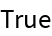#Function Repository Resource:

# ComplementaryAngleDegree

Compute the geometric complement to a given angle using degrees

Contributed by: Paco Jain (Wolfram Research)
 ResourceFunction["ComplementaryAngleDegree"][θ] gives the complement to the angle of θ in degrees.

## Details and Options

ResourceFunction["ComplementaryAngleDegree"] takes all angles to be measured in degrees.
The complement of an angle θ is defined to be the angle which sums with θ to form a right angle. In other words, 90°-θ.

## Examples

### Basic Examples

Compute the complement of an angle:

 In:=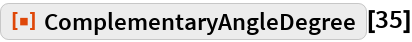Out=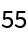### Scope

ComplementaryAngleDegree works directly on Quantity expressions with units of “AngularDegrees”:

 In:=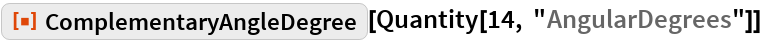Out=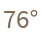ComplementaryAngleDegree works directly on DMS lists of the type returned by DMSList:

 In:=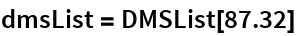Out=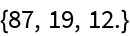In:=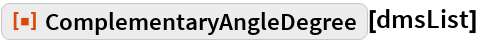Out=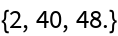ComplementaryAngleDegree works directly on DMS strings of the type returned by DMSString:

 In:=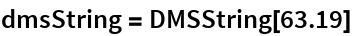Out=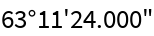In:=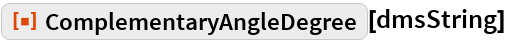Out=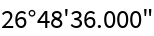### Properties and Relations

ComplementaryAngleDegree will remain unevaluated for non-numeric input:

 In:=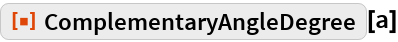Out=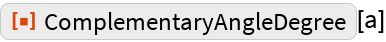ComplementaryAngleDegree will return a negative result if the input angle is greater than 90°:

 In:=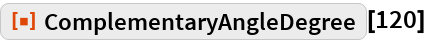Out=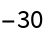For negative input, ComplementaryAngleDegree returns a result greater than 90°:

 In:=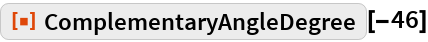Out=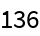For numeric input, ComplementaryAngleDegree is its own inverse:

 In:=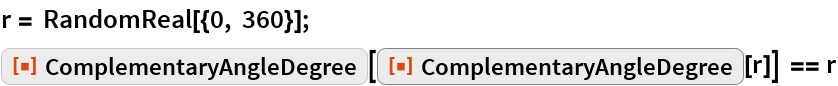Out=